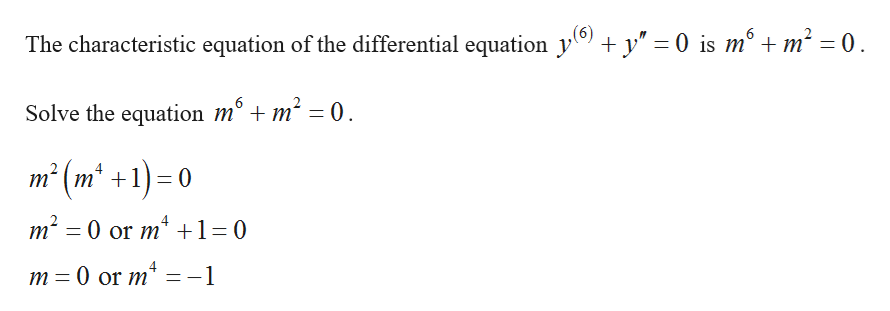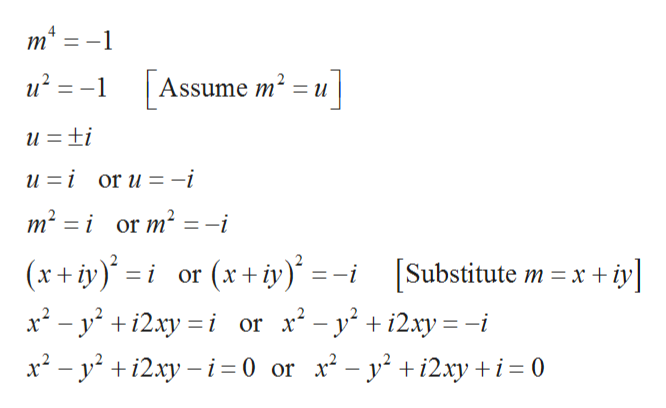# Find the general solutions to the given differential equations:y(6)+y"=0y(3)+3y"+3y'+y=0

Question
35 views

Find the general solutions to the given differential equations:

• y(6)+y"=0
• y(3)+3y"+3y'+y=0
check_circle

Step 1

The differential equations are given by,

Step 2

Obtain the characteristic equation.help_outlineImage Transcriptionclose(6) The characteristic equation of the differential equation y y" 0 is mo m n2 = 0 6 Solve the equation m° + m' m2 (m2 +1)0 m 0 or m* 1 0 0 or m m fullscreen
Step 3

Solve the equation m 4&...help_outlineImage Transcriptionclosem2 и? 3 -1 Assume m? 3Dи] и %3Dti и 3і or u %3 -i m2 ior m2 = - (x+iy)iSubstitute m xiy (x+byi x* - у +12ху —і or x*- y* + i2xy%3D-i х? - у? +12ху — і%3D0 or x* - y? +i2ку +і-0 i or х fullscreen

### Want to see the full answer?

See Solution

#### Want to see this answer and more?

Solutions are written by subject experts who are available 24/7. Questions are typically answered within 1 hour.*

See Solution
*Response times may vary by subject and question.
Tagged in

### Math